﻿ 小波阈值降噪在手写数字识别图像预处理中的应用

# 小波阈值降噪在手写数字识别图像预处理中的应用Application of Wavelet Threshold Denoising in Image Preprocessing of Handwritten Numeral Recognition

Abstract: Image denoising is an important part of image preprocessing of handwritten numeral recognition. For the shortcomings of traditional denoising methods, a wavelet threshold denoising method based on improved semisoft threshold function is proposed. On the one hand, this method can effectively reduce the self-oscillation of hard threshold function and the fuzzy distortion of soft threshold function. On the other hand, compared with the traditional semisoft threshold function, the transition near the threshold is smoother. Combining the vortex search algorithm and the generalized cross validation criterion, the hierarchical threshold is determined adaptively. Simulation results verify the feasibility and effectiveness of this method. Compared with the traditional wavelet threshold denoising methods, the improved method can significantly increase the accuracy of handwritten numeral recognition.

1. 引言

HNR系统主要分为预处理、特征提取、分类判别等模块。其中，预处理是指将原始的图像转换成识别器所能接受的二进制形式，它的主要目的是滤除输入图像中的噪声，压缩图像的冗余信息，为下一步的识别工作奠定基础 。预处理的结果会显著影响整个系统的性能。也就是说，预处理得到的图像质量越好，后续的特征提取和分类判别的效率越高，准确率也越高。

2. 图像的噪声模型

$P\left(x\right)=\frac{1}{\sqrt{2\pi }\sigma }{\text{e}}^{-{\left(x-\mu \right)}^{2}/2{\sigma }^{2}}$ (1)

$P\left(x\right)=\left\{\begin{array}{l}{P}_{a}\text{ }\text{ }\text{ }\text{ }\text{ }\text{ }\text{ }\text{ }\text{ }\text{ }\text{ }\text{ }\text{ }\text{ }\text{ }\text{ }\text{ }\text{ }\text{ }\text{ }\text{ }\text{ }\text{ }\text{ }\text{ }x=a\\ {P}_{b}\text{ }\text{ }\text{ }\text{ }\text{ }\text{ }\text{ }\text{ }\text{ }\text{ }\text{ }\text{ }\text{ }\text{ }\text{ }\text{ }\text{ }\text{ }\text{ }\text{ }\text{ }\text{ }\text{ }\text{ }\text{ }x=b\\ 0\text{ }\text{ }\text{ }\text{ }\text{ }\text{ }\text{ }\text{ }\text{ }\text{ }\text{ }\text{ }\text{ }\text{ }\text{ }\text{ }\text{ }\text{ }\text{ }\text{ }\text{ }\text{ }\text{ }\text{ }\text{ }\text{ }\text{ }\text{ }其他\end{array}$ (2)

3. 基于改进小波阈值函数的降噪方法

$h\left(n\right)=s\left(n\right)+\epsilon \left(n\right)$ (3)

$h\left(n\right)$ 作离散小波变换，得到

$\stackrel{˜}{h}\left(m,n\right)=\stackrel{˜}{s}\left(m,n\right)+\stackrel{˜}{\epsilon }\left(m,n\right)$ (4)Figure 1. Procedures of wavelet threshold denoising

${\stackrel{^}{\stackrel{˜}{h}}}_{m,n}=\left\{\begin{array}{l}0\text{ }\text{ }\text{ }\text{ }\text{ }\text{ }\text{ }\text{ }\text{ }\text{ }\text{ }\text{ }\text{ }\text{ }\text{ }\text{ }\text{ }\text{ }\text{ }\text{ }\text{ }\text{ }\text{ }\text{ }\text{ }\text{ }\text{ }\text{ }\text{ }\text{ }\text{ }\text{ }\text{ }\text{ }\text{ }\text{ }\text{ }\text{ }\text{ }\text{ }\text{ }\text{ }\text{ }\text{ }\text{ }\text{ }\text{ }\text{ }\text{ }\text{ }\text{ }\text{ }\text{ }\text{ }\text{ }\text{ }\text{ }\text{ }\text{ }\text{ }\text{ }\text{ }\text{ }\text{ }\text{ }\text{ }\text{ }\text{ }\text{ }\text{ }\text{ }\text{ }\text{ }\text{ }\text{ }\text{ }\text{ }\text{ }\text{ }\text{ }\text{ }\text{ }\text{ }\text{ }\text{ }\text{ }\text{ }\text{ }\text{ }\text{ }\text{ }\text{ }\text{ }\text{ }\text{ }\text{ }\text{ }\text{ }\text{ }\text{ }\text{ }\text{ }\text{ }\text{ }\text{ }\text{ }\text{ }\text{ }\text{ }\text{ }\text{ }\text{ }\text{ }\text{ }\text{ }\text{ }\text{ }\text{ }\text{ }\text{ }\text{ }\text{ }\text{ }\text{ }\text{ }\text{ }\text{ }\text{ }\text{ }\text{ }\text{ }\text{ }\text{ }\text{ }\text{ }\text{ }\text{ }\text{ }\text{ }\text{ }\text{ }\text{ }\text{ }\text{ }\text{ }\text{ }\text{ }\text{ }\text{ }\text{ }\text{ }|{\stackrel{˜}{h}}_{m,n}|<{T}_{1,m}\\ {\stackrel{˜}{h}}_{m,n}\left[\frac{1}{2}+\frac{1}{2}\mathrm{cos}\left(\frac{|{\stackrel{˜}{h}}_{m,n}|-{T}_{2,m}}{{T}_{2,m}-{T}_{1,m}}\pi \right)\right]\text{ }\text{ }\text{ }\text{ }\text{ }\text{ }\text{ }\text{ }\text{ }\text{ }\text{ }\text{ }\text{ }\text{ }\text{ }{T}_{1,m}\le |{\stackrel{˜}{h}}_{m,n}|\le {T}_{2,m}\\ {\stackrel{˜}{h}}_{m,n}\text{ }\text{ }\text{ }\text{ }\text{ }\text{ }\text{ }\text{ }\text{ }\text{ }\text{ }\text{ }\text{ }\text{ }\text{ }\text{ }\text{ }\text{ }\text{ }\text{ }\text{ }\text{ }\text{ }\text{ }\text{ }\text{ }\text{ }\text{ }\text{ }\text{ }\text{ }\text{ }\text{ }\text{ }\text{ }\text{ }\text{ }\text{ }\text{ }\text{ }\text{ }\text{ }\text{ }\text{ }\text{ }\text{ }\text{ }\text{ }\text{ }\text{ }\text{ }\text{ }\text{ }\text{ }\text{ }\text{ }\text{ }\text{ }\text{ }\text{ }\text{ }\text{ }\text{ }\text{ }\text{ }\text{ }\text{ }\text{ }\text{ }\text{ }\text{ }\text{ }\text{ }\text{ }\text{ }\text{ }\text{ }\text{ }\text{ }\text{ }\text{ }\text{ }\text{ }\text{ }\text{ }\text{ }\text{ }\text{ }\text{ }\text{ }\text{ }\text{ }\text{ }\text{ }\text{ }\text{ }\text{ }\text{ }\text{ }\text{ }\text{ }\text{ }\text{ }\text{ }\text{ }\text{ }\text{ }\text{ }\text{ }\text{ }\text{ }\text{ }\text{ }\text{ }\text{ }\text{ }\text{ }\text{ }\text{ }\text{ }\text{ }\text{ }\text{ }\text{ }\text{ }\text{ }\text{ }\text{ }\text{ }\text{ }\text{ }\text{ }\text{ }\text{ }\text{ }\text{ }\text{ }\text{ }\text{ }\text{ }|{\stackrel{˜}{h}}_{m,n}|>{T}_{2,m}\end{array}$ (5)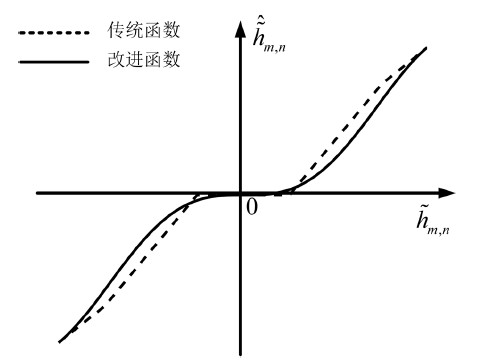Figure 2. Comparison of semisoft threshold functions

$\text{GCV}\left({T}_{1,m},{T}_{2,m}\right)=\frac{N\cdot \underset{n=1}{\overset{N}{\sum }}{\left({\stackrel{˜}{h}}_{m,n}-{\stackrel{^}{\stackrel{˜}{h}}}_{m,n}\right)}^{2}}{{\left(\underset{n=1}{\overset{N}{\sum }}\left(1-|\frac{{\stackrel{^}{\stackrel{˜}{h}}}_{m,n}}{{\stackrel{˜}{h}}_{m,n}}|\right)\right)}^{2}}$ (6)

4. 数值模拟(a)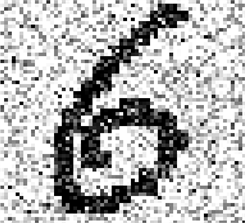(b)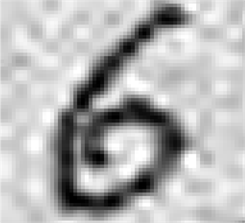(c)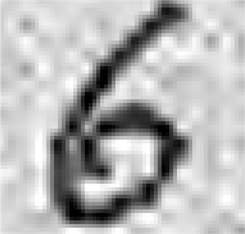(d)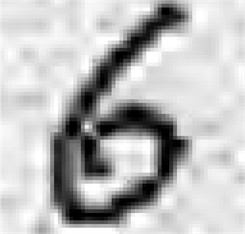(e)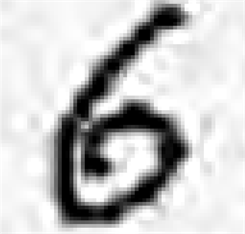(f)

Figure 3. Comparison of denoising effect for different wavelet threshold functions; (a) Original image; (b) Noisy image; (c) Hard threshold denoising; (d) Soft threshold denoising; (e) Semisoft threshold denoising; (f) Improved threshold denoising

$\text{SNR}=10\mathrm{lg}\frac{\underset{n=1}{\overset{N}{\sum }}{h}^{\prime }{\left(n\right)}^{2}}{\underset{n=1}{\overset{N}{\sum }}{\left[{h}^{\prime }\left(n\right)-h\left(n\right)\right]}^{2}}$ (7)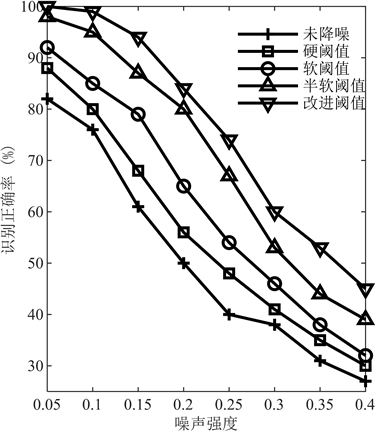Figure 4. Comparison of recognition accuracy for different wavelet threshold functions

5. 结论

 刘辰雨. 基于卷积神经网络的手写数字识别研究与设计[D]: [硕士学位论文]. 成都: 成都理工大学, 2018.

 张猛, 余仲秋, 姚绍文. 手写体数字识别中图像预处理的研究[J]. 微计算机信息, 2008, 22(16): 256-258.

 张翠芳, 杨国为. 手写数字识别中图像预处理技术的研究[J]. 计算机科学与应用, 2016, 6(6): 329-332.

 李志鹏. 基于多尺度几何分析的图像去噪研究[D]: [硕士学位论文]. 南昌: 南昌大学, 2014.

 杨素梅, 陈宏艳, 张闯, 刘素贞. 基于小波原理的电磁声发射信号降噪研究[J]. 计算机工程与设计, 2014, 35(4): 1447-1451.

 张国伟. 基于小波变换的图像去噪方法研究[D]. [硕士学位论文]. 昆明: 昆明理工大学, 2014.

 倪培峰, 胡雄. 一种基于改进阈值函数的小波阈值降噪算法[J]. 测控技术与仪器仪表, 2016, 42(8): 98-104.

 周士贞, 曾向阳, 王璐. 水声信号动态阈值正交匹配追踪降噪方法[J]. 声学技术, 2017, 36(4): 378-382.

 Donoho, D.L. and Johnstone, I.M. (1994) Ideal Spatial Adaption via Wavelet Shrinkage. Biometrika, 81, 425-455.
https://www.jstor.org/stable/2337118
https://doi.org/10.1093/biomet/81.3.425

 Donoho, D.L. (1995) De-Noising by Soft Thresholding. IEEE Transactions on Information Theory, 41, 613-627.
https://dl.acm.org/citation.cfm?id=2274414
https://doi.org/10.1109/18.382009

 魏文畅, 杨俊杰, 蔡建立. 基于小波变换的半软阈值参数算法研究[J]. 计算机工程与应用, 2009, 45(1): 73-76.

 魏振春, 王婿, 徐娟. 基于改进阈值自适应冗余小波的振动信号去噪[J]. 计算机仿真, 2014, 31(11): 192-197.

 贺岩松, 刘程, 徐中明. 基于软阈值和遗传自适应阈值的车辆制动信号的联合小波去噪[J]. 汽车工程, 2014, 36(6): 703-708.

 Dogan, B. and Olmez, T. (2015) A New Metaheu-ristic for Numerical Function Optimization: Vortex Search Algorithm. Information Sciences, 293, 125-145. http://www.sciencedirect.com/science/article/pii/S0020025514008585
https://doi.org/10.1016/j.ins.2014.08.053

 Jansen, M., Malfait, M. and Bultheel, A. (1997) Generalized Cross-Validation for Wavelet Thresholding. Signal Processing, 56, 33-44.
https://www.sciencedirect.com/science/article/pii/S0165168497836213
https://doi.org/10.1016/S0165-1684(97)83621-3

 周鹤峰, 曾新吾. 双面声场反向神经网络声压外推分离[J]. 声学学报, 2018, 43(6): 873-882.

Top